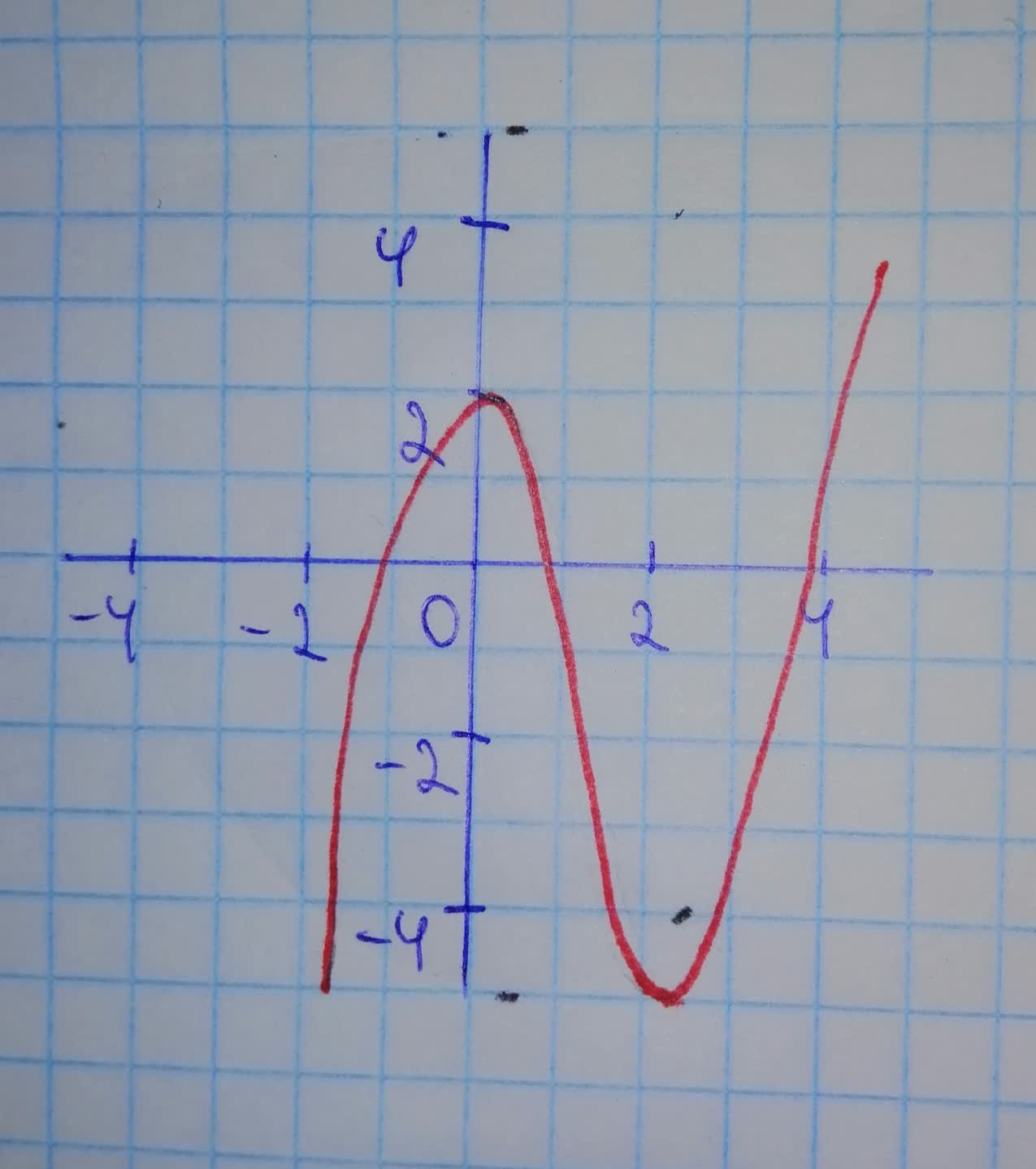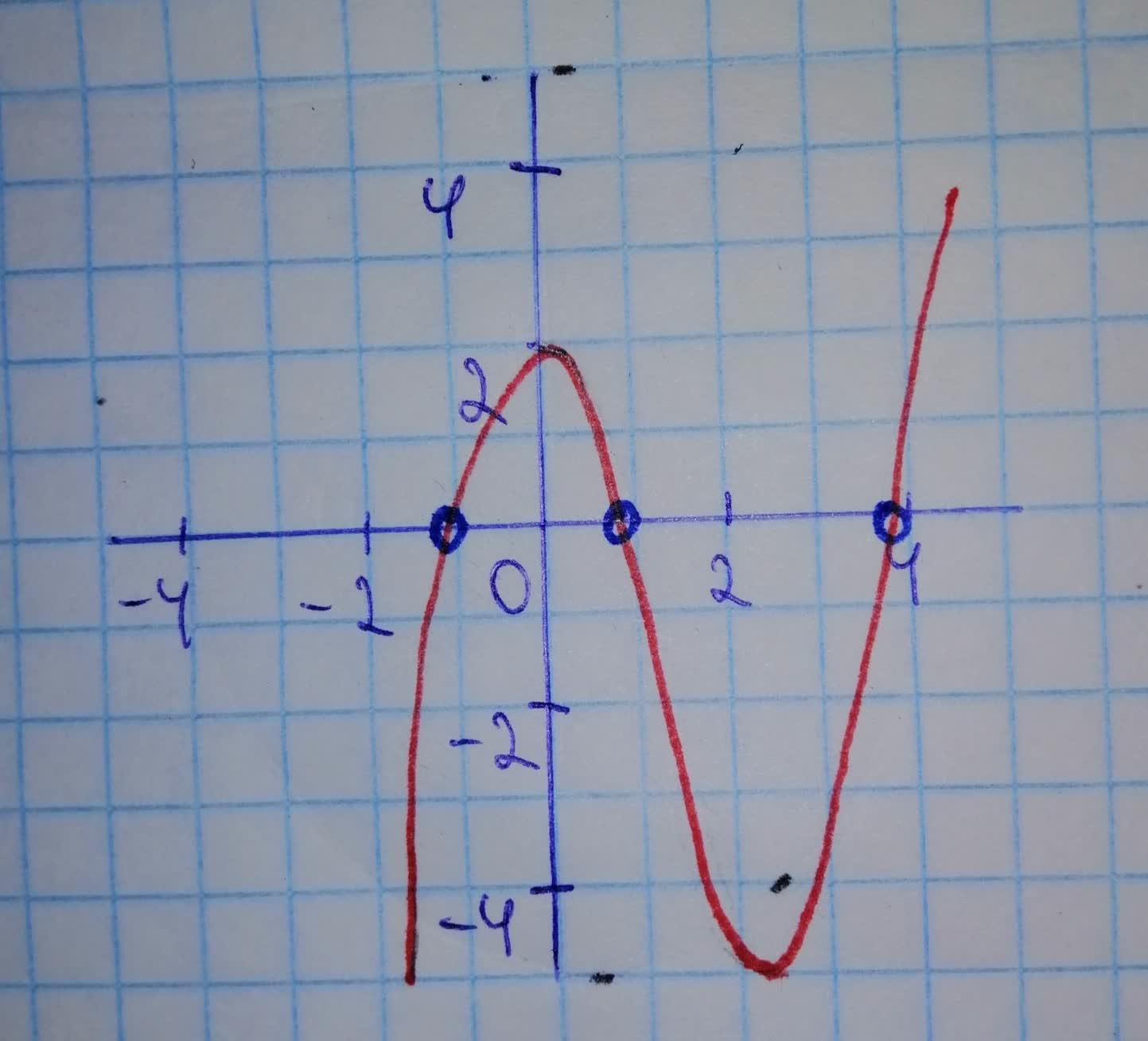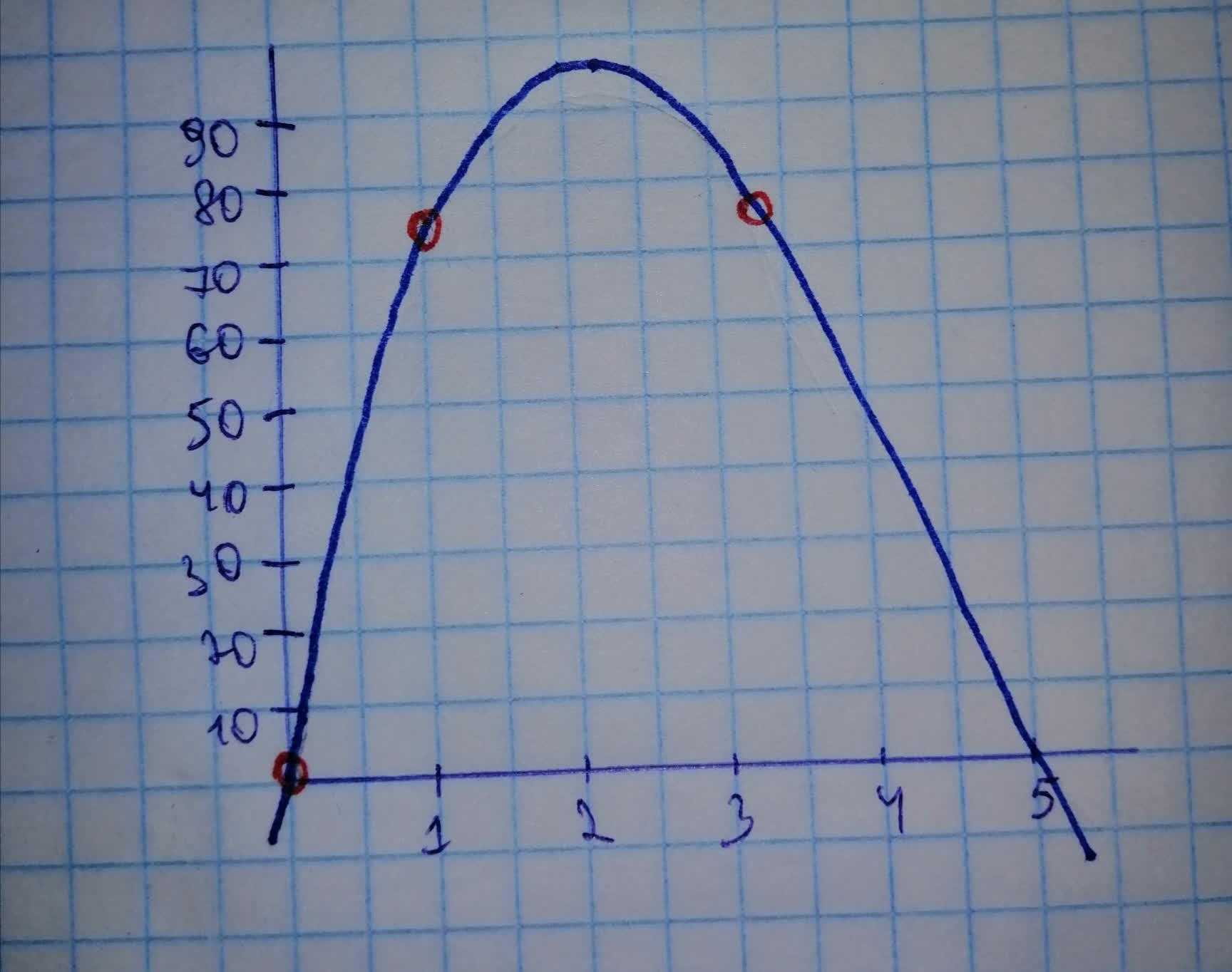# Summarize how graphs can be used to find solutions to polynomial inequalities.dictetzqh 2021-11-20 Answered
Summarize how graphs can be used to find solutions to polynomial inequalities.
You can still ask an expert for help

• Questions are typically answered in as fast as 30 minutes

Solve your problem for the price of one coffee

• Math expert for every subject
• Pay only if we can solve ithuckelig75

Step 1
1. If necessary, rewrite the inequality so that there is a zero on one side.
2. Graph the other side of the inequality.
3. Use your graphing calculator's TRACE function to the exact or approximate values of the x-intercepts.
4. Find the intervals that satisfy the inequality.
5. Write the final result as a solution interval.
Step 2
Example:
${x}^{3}-4{x}^{2}+x+5\le 3$
1. We substract 3 from both sides of the inequality:
${x}^{3}-4{x}^{2}+x+2\le 0$
2. We graph $y={x}^{3}-4{x}^{2}+x+2$Step 3
With the help of the graphing calculator we find the x-intercepts as , rounded to three decimal digits:Step 4
4. We note that the inequality is satisfied on the intervals and .
5. We write the result as a solution interval:

or alternatively
###### Not exactly what you’re looking for?Provere

Step 1
Q2 has been attached here for reference:
$Volume=2{x}^{3}-30{x}^{2}+100x$
$2{x}^{3}-30{x}^{2}+100x\ge 72$Step 2
The x-axis of the graph of a function is the number line over which the function is changing and therefore can be read to find the domain of x for different ranges of the value of $f\left(x\right)$.